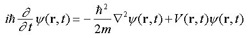A# Schrödinger equation

Schrödinger equation's is an equation in quantum mechanics the solution of which gives the wavefunction (ψ) – a mathematical expression that contains all the information known about a particle. The time-dependent Schrödinger equation describes progressive waves and is applicable to the motion of free particles. The time-independent form describes standing waves.

Schrödinger's equation, named after Erwin Schrödinger, can be solved by the method of eigenvalues devised by Fourier. In this method, any mathematical function is expressed as the sum of an infinite series of other periodic functions. The trick is to find the correct functions that have the right amplitudes so that when added together by superposition they give the desired solution.

The time-dependent Schrödinger equation is:where t is time, r is displacement, m is the mass of the particle, i is the square root of minus one, and h-bar is Planck's constant divided by 2π.